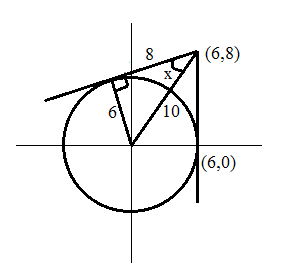0
2376

# CAT Questions On Lines And Angles PDF:

Download CAT Questions On Lines And Angles PDF. Practice important problems on CAT geometry Questions On Lines And Angles with detailed explanations and solutions.

Question 1: In a triangle ABC, Angle B = 60 degres and Angle C = 45 degrees. D is a point on BC such that AD divides BC in the ratio 1:4. Find the value of $\left \lfloor \frac{sin(BAD)}{sin(CAD)} \right \rfloor$.$\left \lfloor \ \right \rfloor$ refers to the greatest integer function less than or equal to x.

a) 1
b) 0
c) 3
d) 5

Question 2: A regular polygon P has 135 diagonals. Find the exterior angle of the polygon P.

a) $18^{\circ}$
b) $20^{\circ}$
c) $25^{\circ}$
d) $30^{\circ}$

Question 3: A circle touches all the three sides of the triangle as shown in the given figure at points P, Q and R respectively. If Angle RPQ is 50 degrees, find the measure of angle BAC (in degrees)?a) 65
b) 70
c) 80
d) 96

Cracku CAT 2020 Course

• 1000 Concept Videos and Solved Question Videos
• 15000 Solved Questions
• 50 MBA Mocks & 45 CAT Sectionals

Question 4: Tangents are drawn from the points (6,8) to the circle $x^2+y^2=36$. Find the angle between the two tangents approximately.

a) $64^{\circ}$
b) $74^{\circ}$
c) $84^{\circ}$
d) $94^{\circ}$

Question 5: A square ABCD has a side of length 3cm. A point E on CD divides CD in such a way that CE:ED = 1:2. AC and BE intersect at P. Find cos(Angle EPC).

a) $\frac{1}{\sqrt{20}}$
b) $\frac{1}{\sqrt{10}}$
c) $\frac{2}{\sqrt{10}}$
d) $\frac{1}{\sqrt{5}}$In triangle ABD,
$\frac{sin(BAD)}{x} =\frac{sin 60}{L}$
$\frac{sin(CAD)}{4x} =\frac{sin 45}{L}$
$4\frac{sin(BAD)}{sin(CAD)} = \frac{\sqrt{3}}{\sqrt{2}} = 1.22$

=> $\frac{sin(BAD)}{sin(CAD)} = 1.22/4 = .305$

$\left \lfloor \frac{sin(BAD)}{sin(CAD)} \right \rfloor$ = $\left \lfloor .305 \right \rfloor$ = 0

Number of diagonals in a regular polygon = $^nC_2 – n$
=> $^nC_2 – n$ = 135
=> n = 18
Exterior angle = $\frac{360}{n}$ = $\frac{360}{18}$ = $20^{\circ}$Angle = ROQ = 2*50 = 100 degrees
Since OR = OQ
Angle ORQ = Angle OQR = (180-100)/2 = 40 degrees
Since line drawn from the centre to the point of contact is perpendicular to the tangent,
Angle ARQ = Angle AQR = 90-40 = 50 degrees
Angle RAQ = 180-50-50 = 80 degreesLet the angle between the tangents be 2x.

The radius of the circle is 6 and the center is (0,0).

=> Distance betweenthe center and point (6,8) is $\sqrt{6^2+8^2}$ = 10

=> Length of the tangent = $\sqrt{10^2-6^2}$ = 8

$\sin x$ = 6/10 = 0.6

$\sin 30^{\circ}$ = 0.5 and $\sin 45^{\circ}$ = $\frac{1}{\sqrt{2}}$ = 0.7

=> x must be around $35^{\circ}$ to $38^{\circ}$

=> 2x must be around $70^{\circ}$ to $76^{\circ}$

Among the options, option B is the closest answer.Angle PEC =$cos^{-1}$(1/$\sqrt{10}$)
Angle EPC = 180-45-$cos^{-1}$(1/$\sqrt{10}$) = 135-$cos^{-1}$(1/$\sqrt{10}$)
cos(Angle EPC) = cos(135-$cos^{-1}$(1/$\sqrt{10})$ ) = cos(135)cos($cos^{-1}$(1/$\sqrt{10}$))+sin135sin($cos^{-1}$(1/$\sqrt{10})$)
cos(Angle EPC) = -$1/\sqrt{20}$+$3/\sqrt{20}$ = $\frac{1}{\sqrt{5}}$